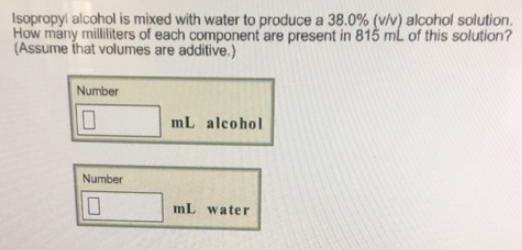# Problem: Isopropyl alcohol is mixed with water to produce a 38.0% (v/v) alcohol solution. How many milliliters of each component are present in 815 mL of this solution? Assume that volumes are additive.)

###### FREE Expert Solution
87% (10 ratings)###### Problem Details

Isopropyl alcohol is mixed with water to produce a 38.0% (v/v) alcohol solution. How many milliliters of each component are present in 815 mL of this solution? Assume that volumes are additive.)